# KSEEB Solutions for Class 7 Maths Chapter 4 Simple Equations Ex 4.4

Students can Download Chapter 4 Simple Equations Ex 4.4, Question and Answers, Notes Pdf, KSEEB Solutions for Class 7 Maths, Karnataka State Board Solutions help you to revise complete Syllabus and score more marks in your examinations.

## Karnataka State Syllabus Class 7 Maths Chapter 4 Simple Equations Ex 4.4

Question 1.
Set up equations and solve them to find the unknown numbers in the following cases :
a) Add 4 to eight times a number; you get 60.
Solution:
Let the unknown number be’x’
8 times a number be 8x
According to the question
8x + 4 = 60
8x = 60 – 4
8x = 56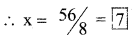∴ The unknown number x be 7

b) One-fifth of a number minus 4 gives 3.
Solution:
Let the unknown number be x
Then according to the question∴ That unknown number be 35.

c) If I take three-fourths of a number and add 3 to it, I get 21.
Solution:
Let the unknown number be x
According to the question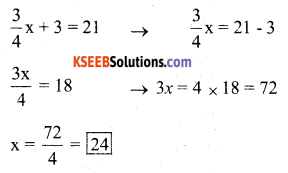∴ That unknown number be 24.d) When I subtracted 11 from twice a number, the result was 15.
Solution:
Let the unknown number be x According to the question
2x – 11 = 15
2x = 15 + 11
2x = 26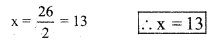∴ That unknown number be 13.

e) Munna subtracts thrice the number of notebooks he has from 50, he finds the result to be 8.
Solution:
Let the unknown number be x
According to the question
50 – 3x = 8
– 3x = 8 – 50Therefore that unknown number be 14.

f) Ibenhal thinks of a number. If she adds 19 to it and divides the sum by 5, she will get 8.
Solution:
Let the unknown number be x
According to the question∴ That unknown number be 21g) Anwar thinks of a number. If he takes away 7 from $$\frac{5}{2}$$ of the number, the result is 23.
Solution:
Let the unknown number be x
According to the question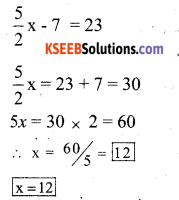That unknown number be 12

Question 2.
Solve the following :
a) The teacher tells the class that the highest marks obtained by a student in her class is twice the lowest marks plus 7. The highest score is 87. What is the lowest score?
Solution:
Let the lowest score be x
According to the question
2x + 7 = 87
2x = 87 – 7 = 80
x = 80/2 = 40 x = 40
∴ The lowest score be 40.b) In an isosceles triangle, the base angles are equal. The vertex angle is 40°. What are the base angles of the triangle? (Remember, the sum of three angles of a triangle is 180°).
Solution:
Let the base angles of an isosceles triangle be x°
According to the question
2x° + 40° = 180°
2x° = 180° – 40°
2x°= 140The base angles are 70° and 70°

c) Sachin scored twice as many runs as Rahul. Together, their runs fell two short of a double century. How many runs did each one score?
Solution:
Let the score of Rahul be ‘x’ runs
According to the question
x + 2x = 198
3x = 198∴ Rahul’s score = x = 66 runs
Sachins score = 2x = 2 × 66 = 132 runs

Question 3.
Solve the following :

i) Irfan says that he has 7 marbles more than five times the marbles Parmit has. Irfan has 37 marbles. How many marbles does Parmit have?
Solution:
Let Parmits marble be x
According to the question
5x + 7 = 37
5x = 37 – 7 = 30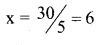∴ Parmits marble be = x = 6

ii) Laxmi’s father is 49 years old. He is 4 years older than three times Laxmi’s age. What is Laxmi’s age?
Solution:
Let the age of Laxmi be ‘x’ years
According to the question
3x + 4 = 49
3x = 49 – 4 = 45
3x = 45
x = 45/3 = 15
∴ Lakshmi’s age is x = 15 years.iii) People of Sundargram planted trees in the village garden. Some of the trees were fruit trees. The number of non-fruit trees were two more than three times the number of fruit trees. What was the number of fruit trees planted if the number of non-fruit trees planted was 77?
Solution:
Let the number of fruit trees be ‘x’
According to the question
3x + 2 = 77
3x = 77 – 2 = 75∴ No. of fruit trees = x = 25

Question 4.
Solve the following riddle :
I am a number, Tell my identity!
Take me seven times over And add a fifty!
To reach a triple century You still need forty!
Solution:
Let the number be ‘x’
According to the question
7x + 50 = 300 – 40
7x + 50 = 260
7x = 260 – 50 = 210∴ That number be x = 30

error: Content is protected !!